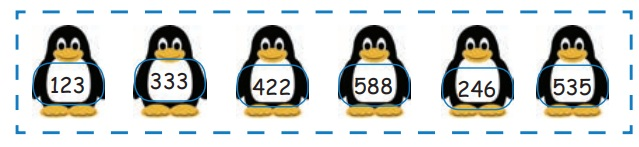Home | | Maths 3rd Std | Ordering

# Ordering

The numbers in ascending and descending order.

Ordering

Consider the numbers 2 and 7. By using these numbers we shall form the greatest and smallest two digit numbers. The two−digit numbers formed using 2 and 7 are 27,72,22,77. (77 is the greatest and 22 is the smallest 2 digit numbers) Similarly, 7, 4 and 8 are given numbers. By using these numbers shall we form the greatest and smallest three digit number.

444, 478, 487, 748, 777, 784, 847, 874 888, and so on

Arrange the given digits from the smallest number to greatest number, we get Ascending order.

478, 487, 748, 784, 847, 874 and so on

Arrange the above from the greatest number to smallest number, we get Descending order.

874, 847, 784, 748, 487,478

888 is the greatest number and 444 is the smallest number.

Practice

1. Form greatest and smallest numbers using the given digits (without repetition of digits)

Number Greatest number Smallest number2. Complete the following number sequence.

111, 222, 333, 444, 555, 666, 777.

150, 155, 160, 165, 170, 175, 180.

210, 310, 410, 510, 610, 710, 810.

333, 433, 533, 633, 733, 833, 933.

3. Find the numbers.

a. 4 Hundreds; 5 Tens; 0 Ones 450

b. 3 Hundreds; 0 Tens; 1 One 301

c. 5 Hundreds; 8 Tens; 9 Ones 589

d. 8 Hundreds; 5 Ones 805

4. Write the number names.

Numeral : Number name

156 : One Hundred and fifty six

340 : Three Hundred and forty

408 : Four Hundred and Eight

696 : Six Hundred and Ninety six

5. Write the place value for the bubbled digits.

a) 1 9 8 Answer: Hundred

b) 9 0 8  Answer: Ones

c) 5 4 3 Answer: Tens

6. Write down the odd and even numbers seperately.

123 333 422 588 246 535a. Odd numbers: 123, 333, 535

b. Even numbers: 422, 588, 246

7. write <, >, = in the box.

105 __ 150 Answer: 105 < 150

419 __ 547 Answer: 419 < 547

394 __ 387 Answer: 394 > 387

761 __ 683 Answer: 761 > 683

660 __ 660 Answer: 660 = 660

983 __ 990 Answer: 983 < 990

8. Write the numbers in ascending and descending order.

326 323 301 356 365 399 308 340

Ascending order : 301, 308, 323, 326, 340, 356, 365, 399

Descending order : 399, 365, 356, 340, 326, 323, 308, 301

9. Using the numerals 6, 8 and 5 only once write the greatest and smallest 3 digit number.

Greatest number : 865

Smallest number : 568

Tags : Numbers | Term 1 Chapter 2 | 3rd Maths , 3rd Maths : Term 1 Unit 2 : Numbers
Study Material, Lecturing Notes, Assignment, Reference, Wiki description explanation, brief detail
3rd Maths : Term 1 Unit 2 : Numbers : Ordering | Numbers | Term 1 Chapter 2 | 3rd Maths